# Why We Need Constructors in C#

## Why We Need Constructors in C#?

In this article, I am going to discuss Why We Need Constructors in C# with Real-time Examples. This is our Part-3 of Constructors. In Part-1 we discussed what exactly a constructor is and in Part-2 we discussed the different types of constructors in C#. In this part, I will demonstrate to you why we need constructors in our class.

##### Why do we need Constructors in C#?

Every class requires a constructor to be present in it if we want to create the instance of that class. Every class contains an implicit constructor if not defined explicitly by the programmer and with the help of that implicit constructor, we can create the instance of that class.

If we don’t define any constructors, then an implicit constructor is there which is provided by the compiler at the time of compilation and using that implicit constructor we can create the instance, then the question is why do we need to define the constructor explicitly again or when do we need to define an explicit constructor in C#?

##### What is the need of Defining a Constructor Explicitly Again?

This is one of the frequently asked interview questions. Let us understand this. Implicit Constructors of a class will initialize variables of a class with the same value even if we create multiple instances of that class.

Let us understand this with an example. Please have a look at the following code. Here, we have a class called First with one variable and then from inside the Main method, we are creating three instances of the First class.

```using System;
namespace ConstructorDemo
{
class First
{
public int x = 100;
}
class Test
{
static void Main(string[] args)
{
First f1 = new First();
First f2 = new First();
First f3 = new First();
Console.WriteLine(\$"{f1.x}   {f2.x}   {f3.x}");
}
}
}
```

Output: 100 100 100

Now, internally, it allocates the memory separately for each instance as shown in the below image. The point that you need to remember, we created three instances and these three instances have a copy of x. And the value is going to be the same for all.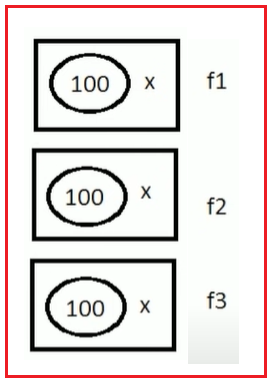This is the problem. Right now, the class contains a constructor i.e. Implicit constructor. And that constructor is going to initialize the variable x with the value 100. So, how many instances we are going to be created, all the instances will be created with the same value.

If we define constructors explicitly with parameters then we will get a chance of initializing the fields or variables of the class with a new value every time we are going to create the instance of that class.

Now, observe the below example, here we have created another class called Second, and this class has a variable called x. Now, the implicit constructor will initialize the x variable with the default value 0 when we created an instance of the Second class and the same for each instance.

```using System;
namespace ConstructorDemo
{
class First
{
public int x = 100;
}
class Second
{
//Initialize with default value
public int x;
}
class Test
{
static void Main(string[] args)
{
First f1 = new First();
First f2 = new First();
First f3 = new First();
Console.WriteLine(\$"{f1.x}   {f2.x}   {f3.x}");

Second s1 = new Second();
Second s2 = new Second();
Second s3 = new Second();
Console.WriteLine(\$"{s1.x}   {s2.x}   {s3.x}");
}
}
}
```
###### Output: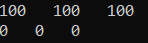Now, I want the x value to be different under the three instances. What value I don’t know, I will come to know the value when I am going to create the instances. Remember one thing whenever we are creating a class means we can reuse the class and we can create the instance whenever and wherever we want. That is code reusability.

So, in the future whenever I am going to create an instance of the class Second, I only need to send the value for x. This is the scenario where we need to go for or defined an explicit parameterized constructor. Let us modify the Second class as follows to add one explicit parameterized constructor to initialize the x variable.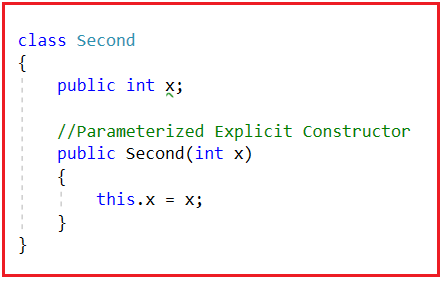Here, this.x refers to the class variable x. See, when I select this.x, automatically the class variable x is highlighted as shown in the below image.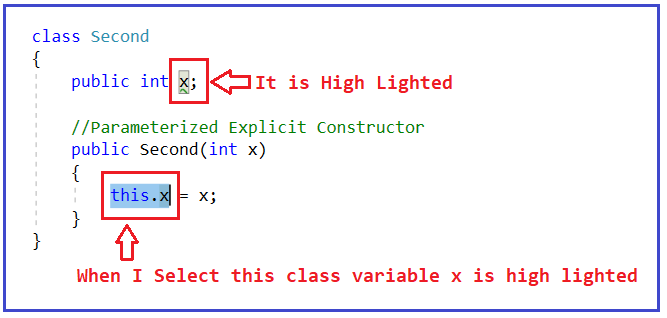And here x refers to the local variable x. See, when I select x, automatically the local variable x is highlighted as shown in the below image.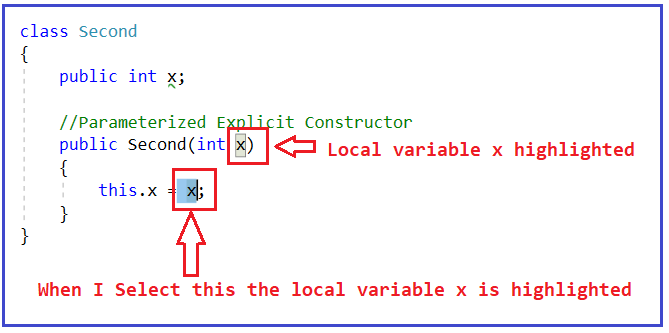This means the local variable x is being assigned to the class variable x. Now, while creating the instance of the Second class, we need to pass a value and that value is stored inside the local variable x. And in this way, while creating multiple instances of the class Second, we can pass different, different values as shown in the below image.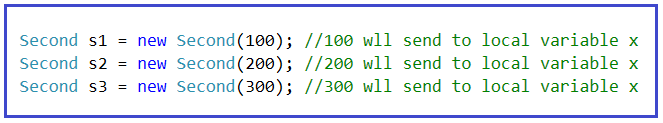The complete example code is given below.

```using System;
namespace ConstructorDemo
{
class First
{
public int x = 100;
}

class Second
{
public int x;

//Parameterized Explicit Constructor
public Second(int x)
{
this.x = x;
}
}

class Test
{
static void Main(string[] args)
{
First f1 = new First();
First f2 = new First();
First f3 = new First();
Console.WriteLine(\$"{f1.x}   {f2.x}   {f3.x}");

Second s1 = new Second(100); //100 wll send to local variable x
Second s2 = new Second(200); //200 wll send to local variable x
Second s3 = new Second(300); //300 wll send to local variable x

Console.WriteLine(\$"{s1.x}   {s2.x}   {s3.x}");
}
}
}
```
###### Output: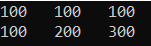The following diagram shows the memory architecture of the above example. Here, for the First class, all the instances contain the same value for x. On the other hand, for class Second, all the instances have a different value and this is possible because of the Explicit Parameterized Constructor in C#.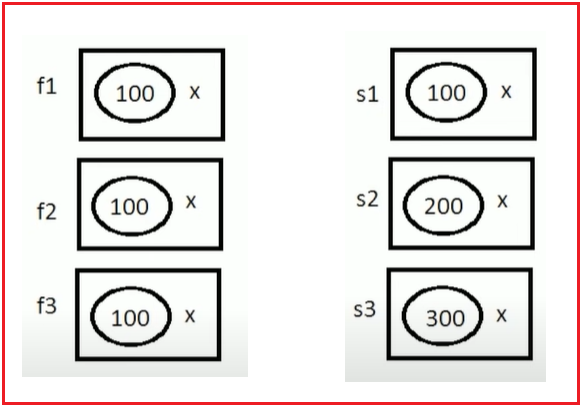When we define a class, first identify whether the class variables require some values to execute and if they are required then define a constructor explicitly and pass values through the constructor, so that every time the instance of the class is created, we get a chance of passing new values.

Note: Generally, every class requires some values for execution, and the values that are required for a class to execute are always sent to that class by using the constructor only.

##### Parameterized Constructor Real-time Example in C#

With the help of a Parameterized constructor, we can initialize each instance of the class with a different set of values. That means using parameterized constructor we can store a different set of values in different objects created in the class. For a better understanding, please have a look at the below example.

```using System;
namespace ConstructorDemo
{
class Employee
{
public int Id, Age;
public bool IsPermanent;

//User Defined Parameterized Constructor
public Employee(int id, int age, string name, string address, bool isPermanent)
{
Id = id;
Age = age;
Name = name;
IsPermanent = isPermanent;
}

public void Display()
{
Console.WriteLine("Employee Id is:  " + Id);
Console.WriteLine("Employee Name is:  " + Age);
Console.WriteLine("Employee Name is:  " + Name);
Console.WriteLine("Is Employee Permanent:  " + IsPermanent);
}
}

class Program
{
static void Main(string[] args)
{
Employee e1 = new Employee(101, 30, "Pranaya", "Mumbai", true);
e1.Display();
Console.WriteLine();

Employee e2 = new Employee(101, 28, "Rout", "BBSR", false);
e2.Display();
}
}
}
```
###### Output: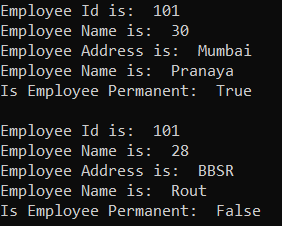##### Copy Constructor Real-time Example in C#

The constructor which takes a parameter of the class type is called a copy constructor. This constructor is used to copy one object’s data into another object. The main purpose of the copy constructor is to initialize a new object (instance) with the values of an existing object (instance). For a better understanding, please have a look at the below example.

```using System;
namespace ConstructorDemo
{
class Employee
{
public int Id, Age;
public bool IsPermanent;

//Parameterized Constructor
public Employee(int id, int age, string name, string address, bool isPermanent)
{
Id = id;
Age = age;
Name = name;
IsPermanent = isPermanent;
}

//Copy Constructor
public Employee(Employee emp)
{
Id = emp.Id;
Age = emp.Age;
Name = emp.Name;
IsPermanent = emp.IsPermanent;
}

public void Display()
{
Console.WriteLine("Employee Id is:  " + Id);
Console.WriteLine("Employee Name is:  " + Age);
Console.WriteLine("Employee Name is:  " + Name);
Console.WriteLine("Is Employee Permanent:  " + IsPermanent);
}
}

class Program
{
static void Main(string[] args)
{
Employee e1 = new Employee(101, 30, "Pranaya", "Mumbai", true);
e1.Display();
Console.WriteLine();

Employee e2 = new Employee(e1);
e2.Display();
}
}
}
```
###### Output: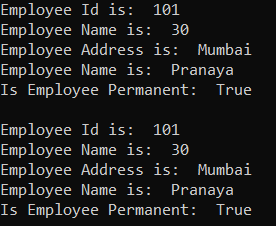##### Static Constructor Real-time Example in C#

The static Constructor in C# will be invoked only once. There is no matter how many instances (objects) of the class are created, it is going to be invoked only once and that is when the class is loaded for the first time.

The static constructor is used to initialize the static fields of the class. You can also write some code inside the static constructor which is going to be executed only once. The static data members in C# are created only once even though we created any number of objects.

```using System;
namespace StaticConstructorDemo
{
class Example
{
int i;
static int j;

//Default Constructor
public Example()
{
Console.WriteLine("Default Constructor Executed");
i = 100;
}

//static Constructor
static Example()
{
Console.WriteLine("Static Constructor Executed");
j = 100;
}
public void Increment()
{
i++;
j++;
}
public void Display()
{
Console.WriteLine("Value of i : " + i);
Console.WriteLine("Value of j : " + j);
}
}
class Test
{
static void Main(string[] args)
{
Example e1 = new Example();
e1.Increment();
e1.Display();
e1.Increment();
e1.Display();
Example e2 = new Example();
e2.Increment();
e2.Display();
e2.Increment();
e2.Display();
}
}
}
```
###### Output: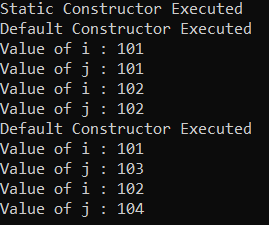In the next article, I am going to discuss Static vs Non-Static Constructors in C# with Examples. Here, in this article, I try to explain Why Do we Need Constructors in C# with Examples. I hope you enjoy this Why Do We Need Constructors in C# with Real-time Examples article. Please give your feedback, suggestions, and questions about this article in the comment section.

### 3 thoughts on “Why We Need Constructors in C#”

1.Guys,
Please give your valuable feedback. And also, give your suggestions about this Why Do we Need Constructors in C# concept. If you have any better examples, you can also put them in the comment section. If you have any key points related to Why Do we Need Constructors in C#, you can also share the same.

2.Slobodan Tajisić

In our first example, does the implicit constructor of the First class initialize the variable x with the value 100, as you wrote (“This is the problem. Right now, the class contains a constructor i.e. Implicit constructor. And that constructor is going to initialize the variable x with the value 100.”)? How does the process of assigning values ​​to variables x take place here? Does the implicit constructor initialize the variable x to 0 and then we assign it the value 100? Or?

3.if we can initialize static variable in non-static constructor then why implicit static- constructor created by compiler? and in static constructor what values initializes ?